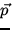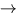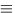## Types of collisions

1.
Inelastic collision:conserved, but not KE. Example - rubber ball on a hard surface (ball deformsinternal elastic PE ).
2.
Perfectly inelastic collision: Two objects stick togetherv1f = v2fvf .conserved, but not KE. Conservation ofgives,
 m1v1i + m2v2i = (m1 + m2)vf. (6)
Example - two lumps of clay.
3.
Elastic collision:and KE are conserved. Example - two billiard balls (no deformations). We have,
 m1v1i + m2v2i = m1v1f + m2v2f, (7)m1v1i2 +m2v2i2 =m1v1f2 +m2v2f2. (8)
By combining these two equations we obtain a third (dependent) equation that tells us that the relative velocity before collision is the negative of the relative velocity after collision:
 v1i - v2i = - (v1f - v2f). (9)Next: Head on Collisions and Up: Collisions and Kinetic Energy Previous: Collisions and Kinetic Energy